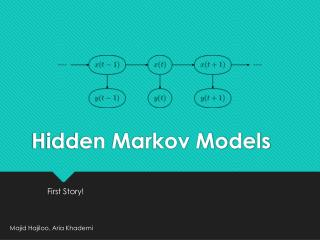DownloadDownload PresentationHidden Markov Models

# Hidden Markov Models

Download Presentation## Hidden Markov Models

- - - - - - - - - - - - - - - - - - - - - - - - - - - E N D - - - - - - - - - - - - - - - - - - - - - - - - - - -
##### Presentation Transcript

1. Hidden Markov Models First Story! Majid Hajiloo, Aria Khademi

2. Outline This lecture is devoted to the problem of inference in probabilistic graphical models (aka Bayesian nets). The goal is for you to: • Practice marginalization and conditioning • Practice Bayes rule • Learn the HMM representation (model) • Learn how to do prediction and filtering using HMMs

3. Assistive Technology Assume you have a little robot that is trying to estimate the posterior probability that you are happy or sad

4. Assistive Technology Given that the robot has observed whether you are: • watching Game of Thrones (w) • sleeping (s) • crying (c) • face booking (f) Let the unknown state be X=h if you’re happy and X=s if you’re sad. Let Y denote the observation, which can be w, s, c or f.

5. Assistive Technology We want to answer queries, such as:

6. Assistive Technology Assume that an expert has compiled the following prior and likelihood models: X Y

7. Assistive Technology But what if instead of an absolute prior, what we have instead is a temporal (transition prior). That is, we assume a dynamical system: • Init Given a history of observations, say we want to compute the posterior distribution that you are happy at step 3. That is, we want to estimate: )

8. Dynamic Model In general, we assume we have an initial distribution , a transition model and an observation model For 2 time steps:

9. Optimal Filtering Our goal is to compute, for all t, the posterior (aka filtering) distribution: We derive a recursion to compute assuming that we have as input . The recursion has two steps: • Prediction • Bayesian update

10. Prediction First, we compute the state prediction:

11. Bayes Update Second, we use Bayes rule to obtain

12. HMM Algorithm Example. Assume: • Prediction: • Bayes Update: if we observe , the Bayes update yields:

13. The END